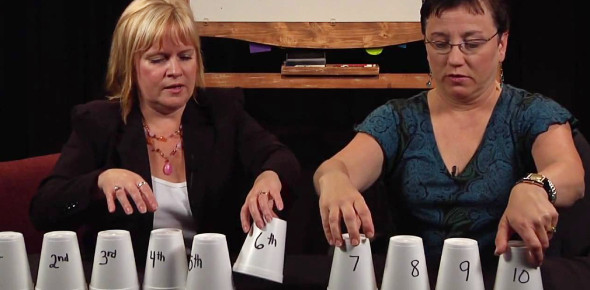# ESL Cardinal & Ordinal Numbers

Approved & Edited by ProProfs Editorial Team
The editorial team at ProProfs Quizzes consists of a select group of subject experts, trivia writers, and quiz masters who have authored over 10,000 quizzes taken by more than 100 million users. This team includes our in-house seasoned quiz moderators and subject matter experts. Our editorial experts, spread across the world, are rigorously trained using our comprehensive guidelines to ensure that you receive the highest quality quizzes.
| By California2011
C
California2011
Community Contributor
Quizzes Created: 5 | Total Attempts: 5,490
Questions: 72 | Attempts: 3,175Settings.

• 1.

• 2.

• 3.

• 4.

### 3

Explanation
The answer is "three" because it is the numerical representation of the word "three".

Rate this question:

• 5.

• 6.

• 7.

• 8.

• 9.

• 10.

• 11.

### 10

Explanation
The given question and answer seem to be incomplete or not readable. It is not clear what the question is asking for or what the context is. Without further information, it is not possible to provide a meaningful explanation for the given answer.

Rate this question:

• 12.

• 13.

• 14.

• 15.

• 16.

• 17.

• 18.

• 19.

• 20.

• 21.

• 22.

### 21

Explanation
The given question provides the number "21" in numerical form and asks for the written form of this number. The correct answer "twenty-one" accurately represents the written form of the number 21.

Rate this question:

• 23.

### 30

Explanation
The answer is "thirty" because it is the written form of the number "30".

Rate this question:

• 24.

### 31

Explanation
The number 31 is written as "thirty-one" in words.

Rate this question:

• 25.

### 40

Explanation
The given answer "forty" is the written form of the number 40.

Rate this question:

• 26.

• 27.

• 28.

• 29.

• 30.

• 31.

### 100

Explanation
The question asks for an explanation of the given correct answer, which is "one hundred". The number 100 is commonly represented as "one hundred" in English. This is because the number 100 consists of one set of 100 units. Therefore, "one hundred" accurately represents the numerical value of 100.

Rate this question:

• 32.

### 1,000

Explanation
The given answer, "one thousand," is the correct answer because it accurately represents the numerical value of the number 1,000. It is a commonly used term to describe this specific quantity and is easily understood by English speakers.

Rate this question:

• 33.

### 10,000

Explanation
The number 10,000 is equivalent to ten thousand.

Rate this question:

• 34.

### 100,000

Explanation
The given answer, "one hundred thousand," is the written form of the number 100,000. In the decimal system, numbers are represented using place values, with each digit's position indicating its value. In this case, the digit 1 is in the hundred thousands place, representing one hundred thousand. Therefore, the answer is correct.

Rate this question:

• 35.

### 1,000,000

Explanation
The given answer "one million" is the word representation of the number 1,000,000. In the English language, numbers can be written in both numeric and word form. In this case, "one million" is the word form of the number 1,000,000, which is composed of the numeral "one" and the unit "million." This is a commonly used way to express large numbers in a more easily understandable format.

Rate this question:

• 36.

• 37.

• 38.

• 39.

### 4th

Explanation
The given answer "fourth" is correct because the question is asking for the ordinal number corresponding to the word "4th." In the English language, "fourth" is the ordinal number for the cardinal number "4."

Rate this question:

• 40.

### 5th

Explanation
The correct answer is "fifth" because it is the ordinal number that represents the position of an item in a series when there are a total of five items.

Rate this question:

• 41.

### 6th

Explanation
The given question is incomplete and does not provide any context or information to determine the meaning or purpose of the word "sixth". Therefore, it is not possible to provide a meaningful explanation for the given correct answer.

Rate this question:

• 42.

### 7th

Explanation
The given answer "seventh" is the correct answer because it corresponds to the ordinal number for the position of "7th" in a sequence. The word "seventh" is derived from the cardinal number "seven" and is commonly used to indicate the position of something in a series or order.

Rate this question:

• 43.

• 44.

• 45.

• 46.

• 47.

• 48.

### 13th

Explanation
The answer "thirteenth" is correct because it is the word that corresponds to the numerical value "13th". In English, ordinal numbers are used to indicate the position of something in a series or sequence. "Thirteenth" is the ordinal form of the cardinal number "thirteen" and represents the position of being the 13th in a series.

Rate this question:

• 49.

### 14th

Explanation
The word "fourteenth" is the ordinal form of the number 14. It is used to indicate the position of something in a series or sequence. In this case, "fourteenth" is the correct answer because it corresponds to the number 14, which is the ordinal form of the number 14.

Rate this question:

• 50.

### 15th

Related TopicsBack to top